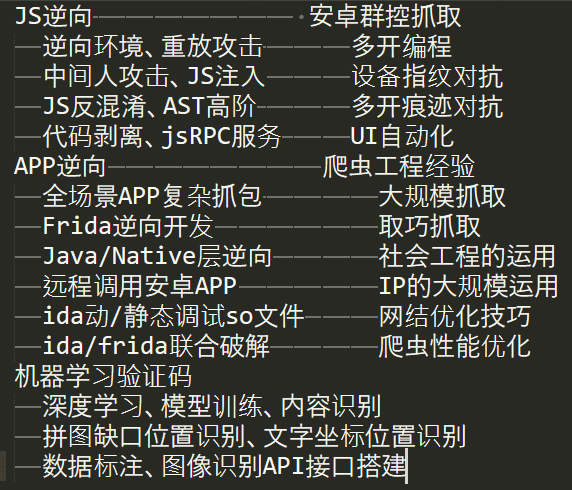Series创建,name,index(索引),values(值),dtype等属性

2020-11-17 144赞 老董笔记

数组：

数组的概念可以看做是一群数据的集合，有点类似python的列表、元组，但是并不相同。在pandas中提到数组一般指的是ndarray(numpy中的数组)

Series数据结构

1、Series是一个一维数组(带有索引,索引可以为数值也可以为标签(比如a、b、c))，能够保存任何数据类型（整数、字符串、浮点数、Python对象等）。

2、Series和DataFrame都有索引，只不过DataFrame同时有列和行索引。

3、Series相比ndarray多了一个索引，相当于一位数组+索引。

4、Series和dict相比，Series更像有顺序的字典，其中字典的key就是索引，索引可以是数字也可以是字母。

5、Series有索引，也有name属性(可以理解为excel的一列的表头)

6、只看Series的值，就是ndarray数组

Series创建(通过字典、数组、标量)：

1、通过字典创建，字典的键就是索引，值就是值。也可以人为指定了index(索引)，如果指定的index没有对应的值，那么就会用缺失值(空值)替代。

# -*- coding: utf-8 -*-

import pandas as pd

# 通过dict创建
d = {'A': 1, 'B': 0, 'C': 2}
s = pd.Series(d)
print(s)
print('==================')
s2 = pd.Series(d, index=['A', 'B', 'c', 'd'])
print(s2)

A    1
B    0
C    2
dtype: int64
==================
A    1.0
B    0.0
c    NaN
d    NaN
dtype: float64

2、通过数组(np数组、列表、元组等)创建

# -*- coding: utf-8 -*-

import pandas as pd
import numpy as np

# 通过数组创建
arr = np.random.rand(5)
s = pd.Series(arr)
print(s)
print('============')
s2 = pd.Series(arr,index=['a','b',3,4,1])
print(s2)
0    0.543199
1    0.261755
2    0.464825
3    0.119778
4    0.436607
dtype: float64
============
a    0.543199
b    0.261755
3    0.464825
4    0.119778
1    0.436607
dtype: float64

3、通过标量创建

index索引值可以出现相同的，但是应避免这样

# -*- coding: utf-8 -*-

import pandas as pd
import numpy as np

# 通过标量创建
s = pd.Series(5., index=['a', 'b', 'c', 'd', 'e'])
print(s)
a    5.0
b    5.0
c    5.0
d    5.0
e    5.0
dtype: float64

name属性及rename方法：

1、Series的name属性(相当于excel的一列添加了表头名)

2、rename方法修改name

# -*- coding: utf-8 -*-

import pandas as pd
import numpy as np

# 通过标量创建
s = pd.Series(5., index=['a', 'b', 'c', 'd', 'e'],name = '高度')
print(s)
print('-----------')
s.name = '长度'
print(s)
print('-----------')
s = s.rename('宽度')
print(s)
a    5.0
b    5.0
c    5.0
d    5.0
e    5.0
Name: 高度, dtype: float64
-----------
a    5.0
b    5.0
c    5.0
d    5.0
e    5.0
Name: 长度, dtype: float64
-----------
a    5.0
b    5.0
c    5.0
d    5.0
e    5.0
Name: 宽度, dtype: float64

Series的index属性(获取索引)、values属性(获取值)

# -*- coding: utf-8 -*-

import numpy as np
import pandas as pd

arr = np.random.rand(3)
s = pd.Series(arr)
print('s的索引',s.index,type(s.index))
print('把s索引变为列表',list(s.index))
print('s的值',s.values,type(s.values))
print('把s的值变为列表',list(s.values))
s的索引 RangeIndex(start=0, stop=3, step=1) <class 'pandas.core.indexes.range.RangeIndex'>

s的值 [0.86401824 0.86229577 0.4742213 ] <class 'numpy.ndarray'>

我们来综合看一下series的常见属性，其中dtype和dtypes相对比较复杂，我们后面会专门解释：

 1 axes 返回行轴标签列表。 2 dtype 返回对象的数据类型(dtype)。 3 dtypes 返回对象的数据类型(dtypes)。 4 empty 如果系列为空，则返回True。 5 ndim 返回底层数据的维数，默认定义：1。 6 size 返回基础数据中的元素数。 7 values 将系列作为ndarray返回。 8 head() 返回前n行。 9 tail() 返回最后n行。文章评论

Series创建,name,index(索引),values(值),dtype等属性文章写得不错，值得赞赏
• 个人微信扫码工作时间

周一至周日 9:00-21:00

• 如果你觉得本站很棒，可以通过扫码支付打赏哦！

•微信收款码
•支付宝收款码